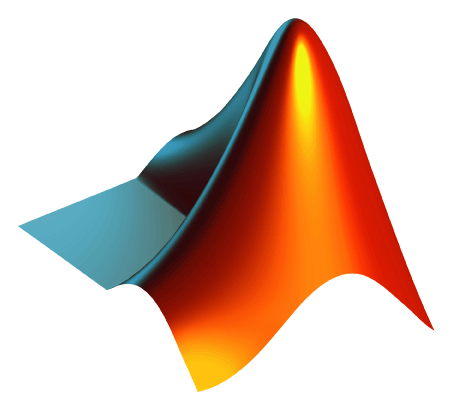# OSE3043 - Analytical Methods of Photonics

Applications-oriented course on analytical concepts prevalent in optics and engineering integrating Matlab as a computational support tool.Credits: 3 hours

Prerequisite:  MAP 2302 Differential Equations

Detailed course description:

Analytical and Coding Methods of Optics will expose the students to common analytical concepts used extensively in optics, physics, and other engineering disciplines. With a focus on applications, this class is designed to teach the students these concepts through relevant optical and engineering examples. The homework will have a required Matlab component so the students, throughout the entirety of this course will gain an intuitive understanding for computer coding and Matlab specifically. This analytical methods class will extensively cover matrix and vector manipulations, solutions of linear systems, eigenvalues and eigenvectors, geometric transformations, and complex analysis.

Learning Outcomes:

At the end of this class the students will be able to tackle advanced concepts in matrix and vector problems that arise throughout the optics and engineering curriculum. They will be proficient users of Matlab and other similar computer coding techniques and be able to tackle advanced computation problems; they will have gained enough intuition with Matlab so that they will be able to apply techniques that are more advanced if and when needed. The students will be able to transform from one bases to another and more importantly why the transformations are important and when to use them and the right ones to use for a specific problem. This class will help with the reinforcement of Analytical concepts that any optical scientist or general engineer should know.

Topics:

1. Introduction to Matlab
• Variable definitions, building a vector, building a matrix
• Functions, operations, loops
• Plotting tools
• Animations
2. Linear Systems
• Matrix Algebra
• Matrix Manipulation
• Systems of linear equations
• Determinants
• Diagonalization
• Eigenvalues and Eigenvectors
3. Vector Analysis
• Dot, Cross, Triple Products, Differentiation of vectors
• Line integrals, Green theorem
• Solid angle calculation and integrating over a surface
• Divergence, Curl, Stokes’ theorem
4. Complex Analysis
5. Transformation spaces
• Bessel
• Laplace
• Fourier

Textbook:

Matlab: A Practical Introduction to Programming and Problem Solving 4th edition, Stormy Attaway

Recommended References:

Advanced Engineering Mathematics 10th edition, Erwin Kreyszig
Matlab for Engineers 4th edition, Holly Moore

Syllabi